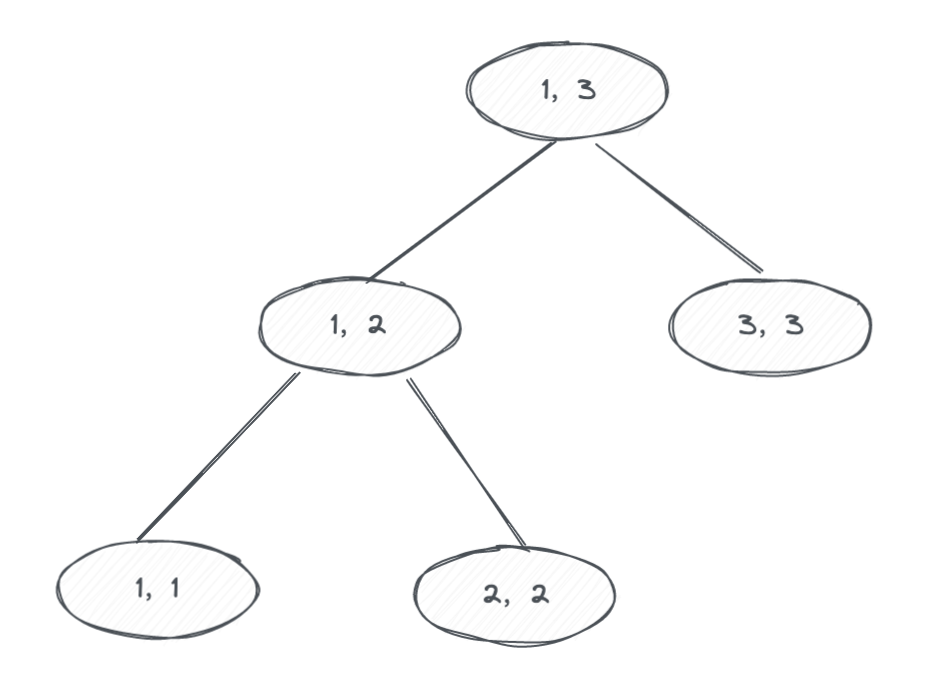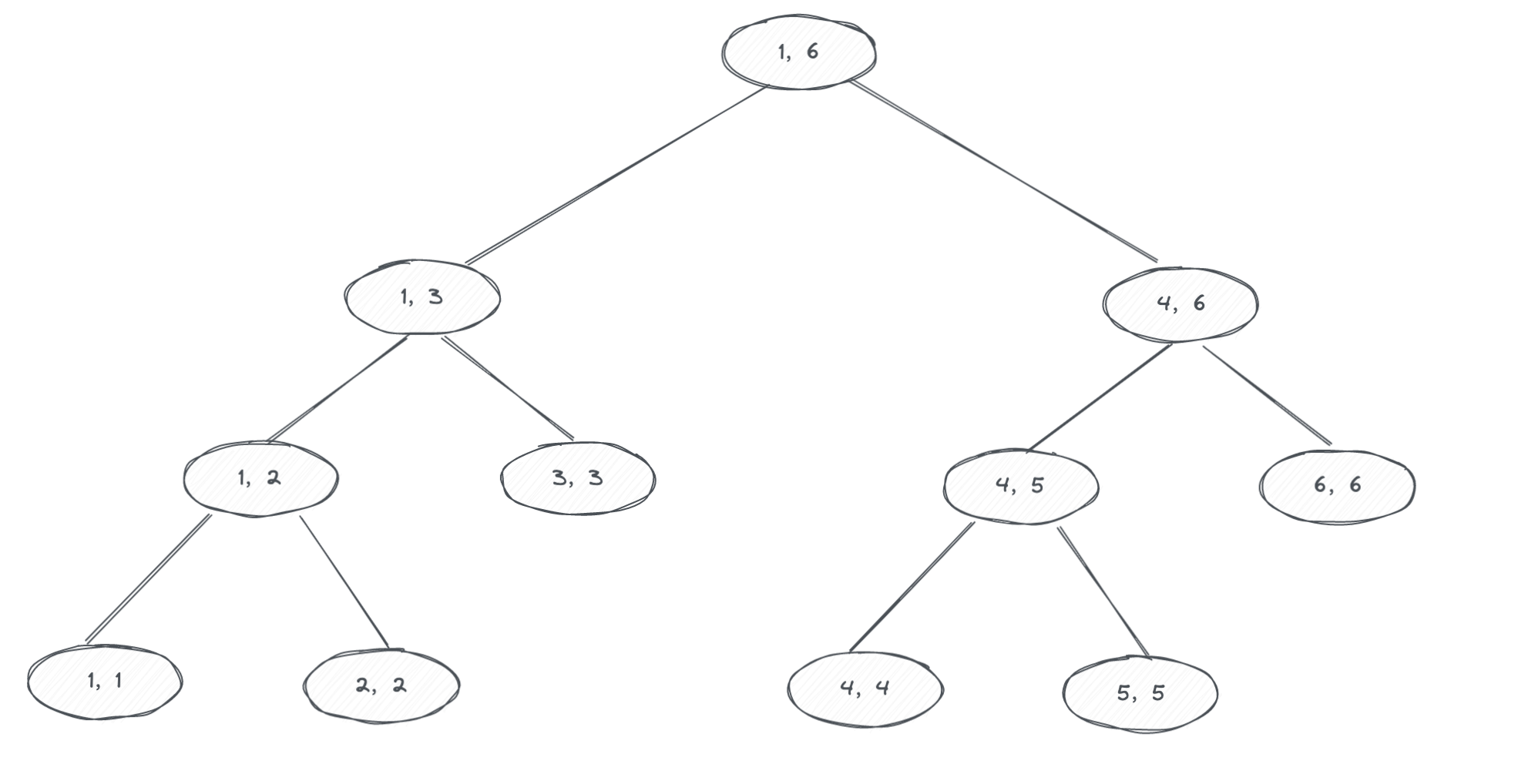### 什么是线段树？### 怎么实现线段树？

#### 构建

build(idx, left, right) {
if(left === right) {
segment[idx] = baseNums[left]
}
mid = (left + right) >> 1
build(idx * 2, left, mid)
build(idx * 2 + 1, mid + 1, right)

// 这里是求和，可以根据要求储存最小值、最大值……
segment[idx] = segment[idx * 2] + segment[idx * 2 + 1]
}


#### 更新

update(target, value, idx, left, right) {
if(left === right) {
segment[idx] = value
}

mid = (left + right) >> 1
if(target <= mid) {
change(target, value,idx * 2, left, mid)
} else {
change(target, value, idx * 2 + 1, mid + 1, right)
}
// 这里是求和，可以根据要求储存最小值、最大值……
segment[idx] = segment[idx * 2] + segment[idx * 2 + 1]
}


#### 查询

query(start, end, idx, left, right) {
if(start === left && end === right)
return segment[idx]

mid = (left + right) >> 1
if(end <= mid)
return query(start, end, idx * 2, left, mid)
else if(start > mid)
return query(start, end, idx * 2 + 1, mid + 1, right)
else
// 这里是求和，可以根据要求储存最小值、最大值……
retuen query(start, end, idx * 2, left, mid) +
query(start, end, idx * 2 + 1, mid + 1, right)
}


### 实战演练

leetcode：307. 区域和检索 - 数组可修改

NumArray(int[] nums) 用整数数组 nums 初始化对象
void update(int index, int val) 将 nums[index] 的值 更新 为 val
int sumRange(int left, int right) 返回数组 nums 中索引 left 和索引 right 之间（ 包含 ）的nums元素的 和 （即，nums[left] + nums[left + 1], …, nums[right]）

class NumArray {
private nums: number[];
constructor(nums: number[]) {
this.nums = nums;
}

update(index: number, val: number): void {
this.nums[index] = val
}

sumRange(left: number, right: number): number {
let ans = 0

for (let i = left; i <= right; i++) {
ans += this.nums[i]
}

return ans
}
}class SegmentTree {
private segment: number[]
private baseNums: number[];
constructor(nums: number[]) {
this.baseNums = nums;
this.segment = new Array(nums.length * 4).fill(0)
this.build(1)
}

build(
idx: number,
left: number = 0,
right: number = this.baseNums.length - 1
): void {
if (left === right) {
// 如果是叶子节点直接赋值
this.segment[idx] = this.baseNums[left]
return
}

let mid = (left + right) >> 1
// 分别处理左右子树
this.build(this.leftChild(idx), left, mid)
this.build(this.rightChild(idx), mid + 1, right)

// 父节点存放子节点的和
this.segment[idx] = this.segment[this.leftChild(idx)] + this.segment[this.rightChild(idx)]
}

change(
target: number,
value: number,
idx: number = 1,
left: number = 0,
right: number = this.baseNums.length - 1
): void {
if (left === right) {
this.segment[idx] = value
return
}

let mid = (left + right) >> 1
if (target <= mid)
this.change(target, value, this.leftChild(idx), left, mid)
else
this.change(target, value, this.rightChild(idx), mid + 1, right)

this.segment[idx] = this.segment[this.leftChild(idx)] + this.segment[this.rightChild(idx)]
}

range(
start: number,
end: number,
idx: number = 1,
left: number = 0,
right: number = this.baseNums.length - 1
): number {
if (left === start && right === end) {
return this.segment[idx]
}

let mid = (left + right) >> 1

if (end <= mid)
return this.range(start, end, this.leftChild(idx), mid + 1, right)
else if (start > mid)
return this.range(start, end, this.rightChild(idx), left, mid)
else
return this.range(start, end, this.leftChild(idx), mid + 1, right) +
this.range(start, end, this.rightChild(idx), left, mid)
}

leftChild(idx: number): number {
return idx * 2
}
rightChild(idx: number): number {
return idx * 2 + 1
}
}class NumArray1 {
private segmentTree: SegmentTree;
constructor(nums: number[]) {
this.segmentTree = new SegmentTree(nums)
this.segmentTree.build(1)
}

change(idx: number, value: number): void {
this.segmentTree.change(idx, value)
}

sumRange(left: number, right: number): number {
return this.segmentTree.range(left, right)
}
}


const numArr = new NumArray1([1, 3, 5])

console.log(numArr.sumRange(0, 2)); // 9
numArr.change(1, 2)
console.log(numArr.sumRange(0, 2)); // 8

••